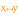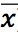# Variance

 Block Group: Statistical Functions Icon:The Variance block calculates the statistical variance of a list of arguments.

The Variance block uses the following equation:

In this equation, s2 is the variance, x is the sample value,is the mean of all x values, and n is the sample size.

For information on variance, see Variance on Wikipedia.

For information on using dataflow blocks, see Dataflow.

For accepted and excluded arguments of Statistical Functions blocks, see Statistical Functions.

## Input/Output Property

The following property of the Variance block can take input and give output.

• input n (number)

input n defines one of the numbers in the set.

## Output Property

The following properties of the Variance block can give output but cannot take input.

• output (number)

output returns the variance of the input values.

## Example

The following image shows an example of the Variance block.